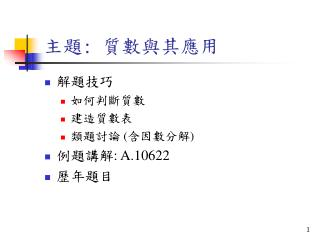Download Presentation# 主題 : 質數與其應用 - PowerPoint PPT PresentationDownload Presentation## 主題 : 質數與其應用

- - - - - - - - - - - - - - - - - - - - - - - - - - - E N D - - - - - - - - - - - - - - - - - - - - - - - - - - -
##### Presentation Transcript

1. 主題: 質數與其應用 • 解題技巧 • 如何判斷質數 • 建造質數表 • 類題討論 (含因數分解) • 例題講解: A.10622 • 歷年題目

2. 質數的定義 • 一個大於等於 2 的數，除了 1 及本身，沒有其他因數，就是質數 • 1 是不是質數依題目規定 (正確而言，不是) • 今天討論假設: 無需處理「大數」

3. 如何判斷質數 • 只要 2 到 n - 1 間的整數都不是 n 的因數，則 n 是質數 • is_prime1(int n) { • for(i = 2 ; i < n ; i++) • if((n % i) == 0) • return false; • return true; • } 最慢，省記憶體

4. 只要 2 到 sqrt(n) 間的整數都不是 n 的因數，則 n 是質數 • is_prime2(int n) { • int m= (int)sqrt(n); • for(i = 2 ; i <= m ; i++) • if((n % i) == 0) • return false; • return true; • } 次慢，省記憶體

5. 假設已建好一範圍在 2 到 n - 1 (n  3) 之間的質數表，存在陣列 prime 中 • prime[i] 代表第 i+1 個質數

6. 只要比 sqrt(n) 小的所有質數都不是 n 的因數，則 n 是質數 (n  3) is_prime3(int n) { int m = (int)sqrt(n); for(i = 0 ; prime[i] <= m ; i++) if((n % prime[i]) == 0) return false; return true; } 最快，浪費記憶體

7. 建造質數表 • 基本法 • 6N  1 法 • Sieve 法（篩法） • 假設我們要求出在 1 ~ range 的範圍內所有的質數

8. 基本法 • 利用判斷質數的方法造表 num_prime = 1; prime = 2; for(i = 3 ; i <= range ; i++) { if(is_prime3(i) == true) { prime[num_prime] = i; num_prime++; } } //最後 prime 中，依序儲存所有質數

9. 6N  1 法 • 只有 6N  1 的數字才需要測試，其他都是 2 or 3 的倍數 num_prime = 2; prime = 2, prime = 3; for(i = 5 ; i <= range ; i++) { if(i%6 == 1 || i%6 == 5) { if(is_prime3(i) == true) { prime[num_prime] = i; num_prime++; } } } //最後 prime 中，依序儲存所有質數

10. Sieve 法 ( 篩法 ) • 不使用除法的建表法 • 對於每個質數來說，它的倍數一定不是質數，所以建立一個大小是 range 的陣列 is_prime，每看到一個質數，就把它的所有倍數砍掉，剩下就是質數 • 優點：快速 • 缺點：範圍有限 (range 不可以太大) • 類題 • 406, 686

11. memset(is_prime, 0, sizeof(is_prime)); //初始設定 is_prime 中所有 element 為 0 for(i = 2; i <= range; i++) { if(is_prime[i] == 0) { for(k = i*2 ; k <= range ; k = k+i) { is_prime[k] = 1; } } } //最後 is_prime 中，index 存 0 是質數

12. Problem: 因數分解 • 解法: 先建質數表，從質數表的第一個質數往後看到超過 sqrt(num)為止，只要除的盡就不斷的除以該質數 • 建立一個 list array (plist) 代表質因數列表 • 建立一個 counter array (ppow) 來代表每個質數的次數 • 類題 • 516、583

13. 60 = 2^2  3^1  5^1 plist ppow 60

14. memset(ppow, 0, sizeof(ppow)); num_pfac = 0; //質因數的個數 for(i = 2 ; i <= (int)sqrt(num) ; i++) { if(is_prime[i] == 0 && num%i == 0) { //使用篩法的質數表 plist[num_pfac] = i; // 放入質因數列表 while(num%i == 0) { ppow[num_pfac]++; //更新次數列表 num = num/i; } num_pfac++; } } if(num_pfac == 0) { // 質數表中找不到因數: 自己就是質數 plist = num; ppow = 1; num_pfac = 1; }

15. Problem: 求因數的個數 • 解法: 先做因數分解，代入求因數個數的公式 • 類題 • 294 因數個數 (q1+1)  (q2+1)  …  (qm+1)

16. Problem: 約分 • 以 為例，直接將分母分子算出，再做除法，有可能 overflow • 解法: 將所有質數代入約分，再把分子乘出 • 類題 • 369，530，160

17. Problem: Goldbach’s conjecture • Goldbach 假說: 所有的偶數都是兩個質數相加的和 • 類題 • 543，686，10168，10311 • Problem 10168: • 給一個偶數 n，找四個質數 p1，p2，p3，p4，使得 n = p1 + p2 + p3 + p4

18. Problem: 孫子點兵 • 有一數除以 3 餘 2，除以 5 餘 3，除以 7 餘 2，這個數字是多少? • 類題 • 498

19. test from 0 to 4 x  2 (mod 3)  x = 3  q1 + 2 x  3 (mod 5)  x = 3  (5  q2 + r2) + 2  r2 = 2，x = 15  q2 + 8 x  2 (mod 7)  x = 15  (7  q3 + r3) + 8  r3 = 1，x = 105  q3 + 23 // m[] 是除數，z[] 是餘數，num_inp 是組數 r = z; q = m; for(i = 1 ; i < num_inp ; i++) { for(j = 0 ; j < m[i] ; j++) if (((j*q+r) % m[i]) == z[i]) break; r = r + j*q; q = q*m[i]; } test from 0 to 6

20. 進階中國餘式定理 • x = (mod M) • M = m  m  …  m[num_inp - 1] • M[i] = M / m[i] • M’[i]  M[i]  1 (mod m[i])

21. 例題講解:A.10622(http://acm.uva.es/p/v106/10622/html) • 給一個 int 允許的數字 x，且該數字絕對值大於等於 2 • 找 p，p 必須是所有可能的 x = b^p 中，最大的一個，b，p 為整數 64 = 8^2 = 4^3 = 2^6 ans: 6

22. Sample input/output 17 1073741824 25 0 Sample input // input 結束 1 30 2 Sample output

23. 資料結構 • #define range 66000 //比 65536 大即可 • int num • 輸入的數字 • int is_prime [range] • 篩法建立的質數表 • int num_pfact • num 的質因數個數 • int ppow[range] • num 因數分解後，每個質因數的次方數

24. 解法 • 建質數表 • 質因數分解 • 決定最大次方數 • 考慮 num 是負數的狀況

25. Program structure construct_is_prime(); //建質數表 while(1) { scanf(“%d”, &num); if(num == 0) break; // input 結束 flag = 0; // 假設 num 為正 if(num < 0) flag = 1; // num 為負，改變 flag factorial(num); //因數分解，只需記次方數 find_max_power(); if(flag == 1) num_is_minus(); output(); } // end of while

26. 決定最大次方數 • 如何決定最大次方數? • n = 129600 = 26 34  52 = (23  32  5)2 • n 的次方數必是質因數次方數的公因數 • 最大次方數為所有的質因數次方數求最大公因數

27. 求公因數 • a, b 兩數求公因數 • 輾轉相除法 int gcd(int a, int b) { int c; if(a < b) swap(a, b); //讓 a > b if(a%b != 0) return gcd(b, a%b); else return b; }

28. 若 num 是負的 • 只有奇數次方才有可能為負數 • 將之前求得的次方數不斷除以二，直到成為奇數為止

29. 歷年題目 • 練習題 • A.10168 Summation of Four Primes • http://acm.uva.es/p/v101/10168.html • A.10622 Perfect Pth Powers • http://acm.uva.es/p/v106/10622.html • A.10140 Prime Distance • http://acm.uva.es/p/v101/10140.html • 挑戰題 • A.10311 Goldbach and Euler • http://acm.uva.es/p/v103/10311.html • 其他歷年題目 • 無